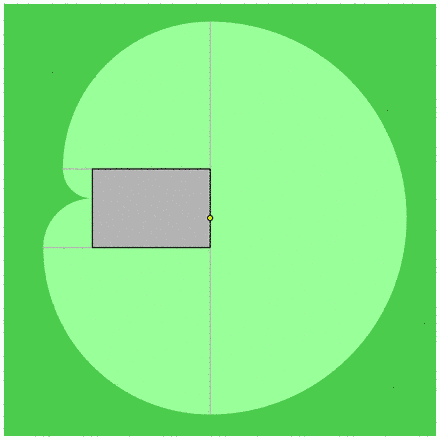Fichier seguin-oa2009.mp (figure 3) — Modifié le 4 Octobre 2009 à 23 h 28

## La chèvre de Monsieur SeguinFigures de l'un des exercices des olympiades académiques de mathématiques (Poitiers, 2009). La chèvre est attachée à l'extrémité d'une chaîne qui est fixée sur le mur extérieur de la bergerie, rectangulaire...
Source
``````

path quart, demi, troisquart, bergerie, bord, terrain;

quart = (reverse fullcircle) scaled 2 cutbefore (0,1);
demi  = (reverse fullcircle) scaled 2 cutbefore (-1,0);
troisquart = fullcircle scaled 2 cutbefore (0,1);
bergerie = (0,0) -- (6,0) -- (6,4) -- (0,4) -- cycle;
terrain := (-5,-11)--(17,-11) -- (17, 11) -- (-5,11) -- cycle;

bord := (quart rotated 90 scaled 4) --
( reverse ( troisquart rotated 90 scaled 10 shifted (6,0) ) ) --
(quart rotated 90 scaled 6 shifted (6,4)) --
cycle;

beginfig(1);

draw bergerie scaled 10;
draw (troisquart rotated 90 scaled 10 shifted (6,0)) scaled 10;
draw (quart rotated 90 scaled 6 shifted (6,4)) scaled 10;
draw (quart rotated 90 scaled 4) scaled 10;

endfig;

beginfig(2);

fill terrain scaled 10 withcolor (0.3,0.8,0.3);

fill bord scaled 10 withcolor (0.6,1,0.6);
% draw bord scaled 10 withcolor (0.6,1,0.6);

draw ((6,0)--(6,10)) scaled 10 withcolor 0.7white;
draw ((-4,0)--(6,0)) scaled 10 withcolor 0.7white;

fill bergerie scaled 10 withcolor 0.7white;
draw bergerie scaled 10;

fill fullcircle scaled 3 shifted ((6,0) scaled 10) withcolor black;
fill fullcircle scaled 2 shifted ((6,0) scaled 10) withcolor (1,1,0);

endfig;

x := 2.5;
bord := (0,x) -- ((quart) rotated   180 scaled (4-x) shifted (0,4)) --
((quart) rotated    90 scaled (10-x) shifted (6,4)) --
((demi)  rotated   -90 scaled 10 shifted (6,4-x)) --
((quart) rotated  -180 scaled (6+x) shifted (6,0))--
((quart) rotated  -270 scaled x shifted (0,0)) -- cycle;

beginfig(3);

x := 2.5;

fill (terrain shifted (0.5,1.4))  scaled 10 withcolor (0.3,0.8,0.3);
fill bord scaled 10 withcolor (0.6,1,0.6);
% draw bord scaled 10 withcolor (0.6,1,0.6);

draw ((6,14-x)--(6,-x-6)) scaled 10 withcolor 0.7white;
draw ((6,0)--(-x,0)) scaled 10 withcolor 0.7white;
draw ((6,4)--(x-4,4)) scaled 10 withcolor 0.7white;

fill bergerie scaled 10 withcolor 0.7white;
draw bergerie scaled 10;

fill fullcircle scaled 3 shifted ((6,4-x) scaled 10) withcolor black;
fill fullcircle scaled 2 shifted ((6,4-x) scaled 10) withcolor (1,1,0);

endfig;

y := 2;
bord := (y,0) -- ((quart) rotated   270 scaled y shifted (0,0)) --
((quart) rotated    180 scaled (y+4) shifted (0,4)) --
((demi)  rotated   0 scaled 10 shifted (6-y,4)) --
((quart) rotated  -90 scaled (10-y) shifted (6,4))--
((quart) rotated  180 scaled (6-y) shifted (6,0)) -- cycle;

beginfig(4);

y := 2;

fill (terrain shifted (-2,5))  scaled 10 withcolor (0.3,0.8,0.3);
fill bord scaled 10 withcolor (0.6,1,0.6);
% draw bord scaled 10 withcolor (0.6,1,0.6);

draw ((16-y,4)--(-4-y,4)) scaled 10 withcolor 0.7white;
draw ((6,4)--(6,y-6)) scaled 10 withcolor 0.7white;
draw ((0,0)--(0,-y)) scaled 10 withcolor 0.7white;

fill bergerie scaled 10 withcolor 0.7white;
draw bergerie scaled 10;

fill fullcircle scaled 3 shifted ((6-y,4) scaled 10) withcolor black;
fill fullcircle scaled 2 shifted ((6-y,4) scaled 10) withcolor (1,1,0);

endfig;

end``````